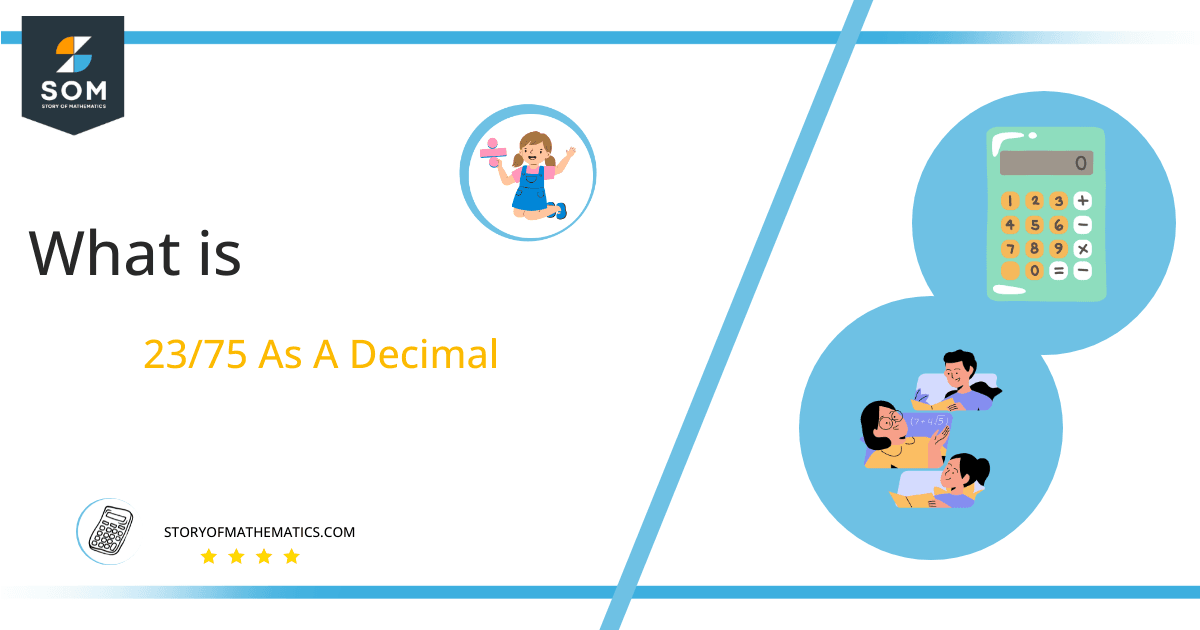# What Is 23/75 as a Decimal + Solution With Free Steps

The fraction 23/75 as a decimal is equal to 0.30666666.

We know that Division is one of the four primary operators of mathematics, and there are two types of divisions. One solves entirely and results in an Integer value, while the other doesn’t translate to completion, producing a Decimal value.Here, we are more interested in the division types that result in a Decimal value, as this can be expressed as a Fraction. We see fractions as a way of showing two numbers having the operation of Division between them that result in a value that lies between two Integers.

Now, we introduce the method used to solve said fraction to decimal conversion, called Long Division, which we will discuss in detail moving forward. So, let’s go through the Solution of fraction 23/75.

## Solution

First, we convert the fraction components, i.e., the numerator and the denominator, and transform them into the division constituents, i.e., the Dividend and the Divisor, respectively.

This can be done as follows:

Dividend = 23

Divisor = 75

Now, we introduce the most important quantity in our division process: the Quotient. The value represents the Solution to our division and can be expressed as having the following relationship with the Division constituents:

Quotient = Dividend $\div$ Divisor = 23 $\div$ 75

This is when we go through the Long Division solution to our problem.Figure 1

## 23/75 Long Division Method

We start solving a problem using the Long Division Method by first taking apart the division’s components and comparing them. As we have 23 and 75, we can see how 23 is Smaller than 75, and to solve this division, we require that 23 be Bigger than 75.

This is done by multiplying the dividend by 10 and checking whether it is bigger than the divisor or not. If so, we calculate the Multiple of the divisor closest to the dividend and subtract it from the Dividend. This produces the Remainder, which we then use as the dividend later.

Now, we begin solving for our dividend 23, which after getting multiplied by 10 becomes 230.

We take this 230 and divide it by 75; this can be done as follows:

230 $\div$ 75 $\approx$ 3

Where:

75 x 3 = 225

This will lead to the generation of a Remainder equal to 230 – 225 = 5. Now this means we have to repeat the process by Converting the 5 into 50 and solving for that:

Still, the dividend is less than the divisor, so we will multiply it by 10 again. For that, we have to add the zero in the quotient. So, by multiplying the dividend by 10 twice in the same step and by adding zero after the decimal point in the quotient, we now have a dividend of 500.

500 $\div$ 75 $\approx$ 6

Where:

75 x 6 = 450

Finally, we have a Quotient generated after combining the pieces of it as 0.306=z, with a Remainder equal to 50.Images/mathematical drawings are created with GeoGebra.